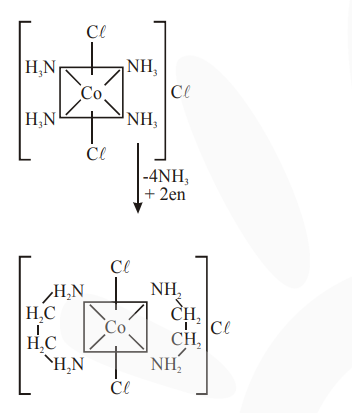# The equivalents of ethylene diamine required to replace the neutral ligands from the coordination sphere of the trans-complex`
Question:

The equivalents of ethylene diamine required to replace the neutral ligands from the coordination sphere of the trans-complex of

$\mathrm{CoCl}_{3} .4 \mathrm{NH}_{3}$ is_________ (Round off to the Nearest Integer).

Solution:

trans $-\mathrm{CoCl}_{3} .4 \mathrm{NH}_{3}$

or

trans- $\left[\mathrm{CO}\left(\mathrm{NH}_{3}\right)_{4} \mathrm{Cl}_{2}\right] \mathrm{C} \ell$As we know that ethylene diamine is a bidentate ligand and ammonia is a mono dentate ligand.

It means overall two ethylene diamine is required to replace the all neutral ligands (four ammonia) from the coordination sphere of this complex